# Tutorial for Spline¶

Background information about B-spline at Wikipedia.

## Splines from fit points¶

Splines can be defined by fit points only, this means the curve goes through all given fit points. AutoCAD and BricsCAD generates required control points and knot values by itself, if only fit points are present.

Create a simple spline:

```doc = ezdxf.new('R2000')

fit_points = [(0, 0, 0), (750, 500, 0), (1750, 500, 0), (2250, 1250, 0)]
msp = doc.modelspace()
spline = msp.add_spline(fit_points)
```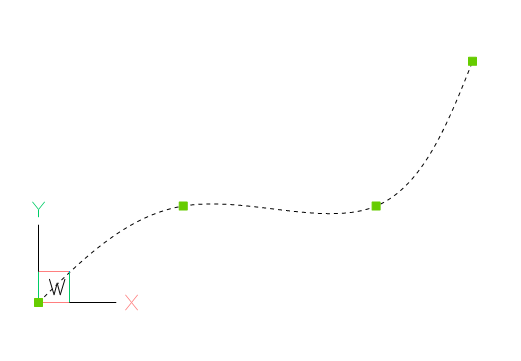Append a fit point to a spline:

```# fit_points, control_points, knots and weights are list-like containers:
spline.fit_points.append((2250, 2500, 0))
```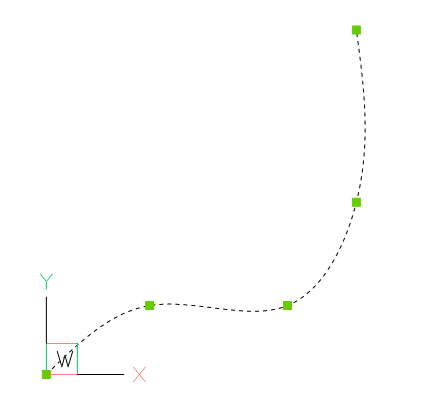You can set additional control points, but if they do not fit the auto-generated AutoCAD values, they will be ignored and don’t mess around with knot values.

Solve problems of incorrect values after editing a spline generated by AutoCAD:

```doc = ezdxf.readfile("AutoCAD_generated.dxf")

msp = doc.modelspace()
spline = msp.query('SPLINE').first

# fit_points, control_points, knots and weights are list-like objects:
spline.fit_points.append((2250, 2500, 0))
```

As far as I have tested, this approach works without complaints from AutoCAD, but for the case of problems remove invalid data:

```# current control points do not match spline defined by fit points
spline.control_points = []

# count of knots is not correct:
# count of knots = count of control points + degree + 1
spline.knots = []

# same for weights, count of weights == count of control points
spline.weights = []
```

## Splines by control points¶

To create splines from fit points is the easiest way to create splines, but this method is also the least accurate, because a spline is defined by control points and knot values, which are generated for the case of a definition by fit points, and the worst fact is that for every given set of fit points exist an infinite number of possible splines as solution.

AutoCAD (and BricsCAD also) uses an proprietary algorithm to generate control points and knot values from fit points, which differs from the well documented Global Curve Interpolation. Therefore splines generated from fit points by ezdxf do not match splines generated by AutoCAD (BricsCAD).

To ensure the same spline geometry for all CAD applications, the spline has to be defined by control points. The method `add_spline_control_frame()` adds a spline trough fit points by calculating the control points by the Global Curve Interpolation algorithm. There is also a low level function `ezdxf.math.global_bspline_interpolation()` which calculates the control points from fit points.

```msp.add_spline_control_frame(fit_points, method='uniform', dxfattribs={'color': 1})
msp.add_spline_control_frame(fit_points, method='chord', dxfattribs={'color': 3})
msp.add_spline_control_frame(fit_points, method='centripetal', dxfattribs={'color': 5})
```
• black curve: AutoCAD/BricsCAD spline generated from fit points
• red curve: spline curve interpolation, “uniform” method
• green curve: spline curve interpolation, “chord” method
• blue curve: spline curve interpolation, “centripetal” method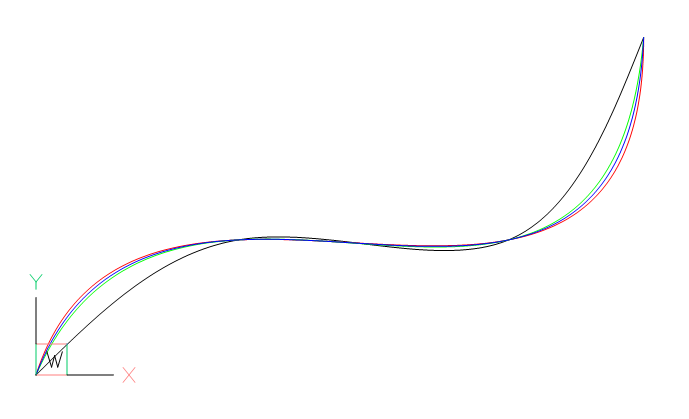### Open Spline¶

Add and open (clamped) spline defined by control points with the method `add_open_spline()`. If no knot values are given, an open uniform knot vector will be generated. A clamped B-spline starts at the first control point and ends at the last control point.

```control_points = [(0, 0, 0), (1250, 1560, 0), (3130, 610, 0), (2250, 1250, 0)]
msp.add_open_spline(control_points)
```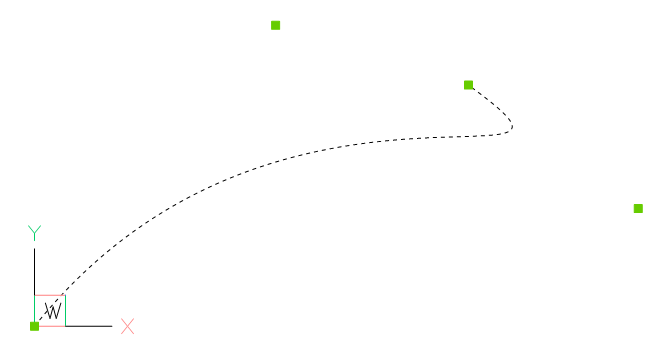### Rational Spline¶

Rational B-splines have a weight for every control point, which can raise or lower the influence of the control point, default weight = `1`, to lower the influence set a weight < `1` to raise the influence set a weight > `1`. The count of weights has to be always equal to the count of control points.

Example to raise the influence of the first control point:

```msp.add_closed_rational_spline(control_points, weights=[3, 1, 1, 1])
```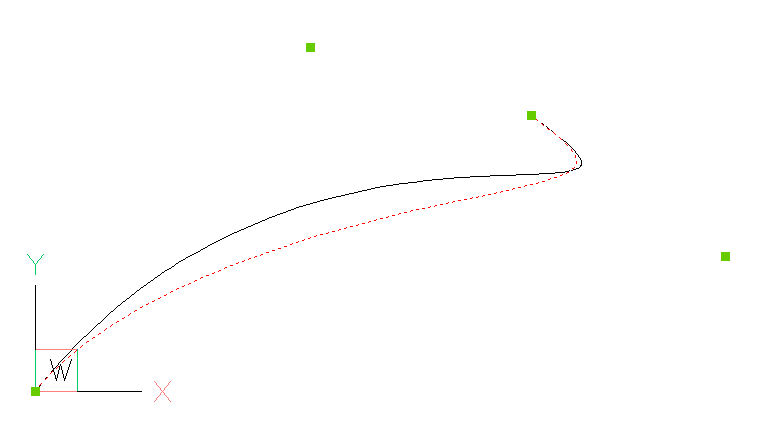## Spline properties¶

Check if spline is a closed curve or close/open spline, for a closed spline the last point is connected to the first point:

```if spline.closed:
# this spline is closed
pass

# close spline
spline.closed = True

# open spline
spline.closed = False
```

Set start- and end tangent for splines defined by fit points:

```spline.dxf.start_tangent = (0, 1, 0)  # in y-axis
spline.dxf.end_tangent = (1, 0, 0)  # in x-axis
```

Get data count as stored in DXF file:

```count = spline.dxf.n_fit_points
count = spline.dxf.n_control_points
count = spline.dxf.n_knots
```

Get data count of real existing data:

```count = spline.fit_point_count
count = spline.control_point_count
count = spline.knot_count
```